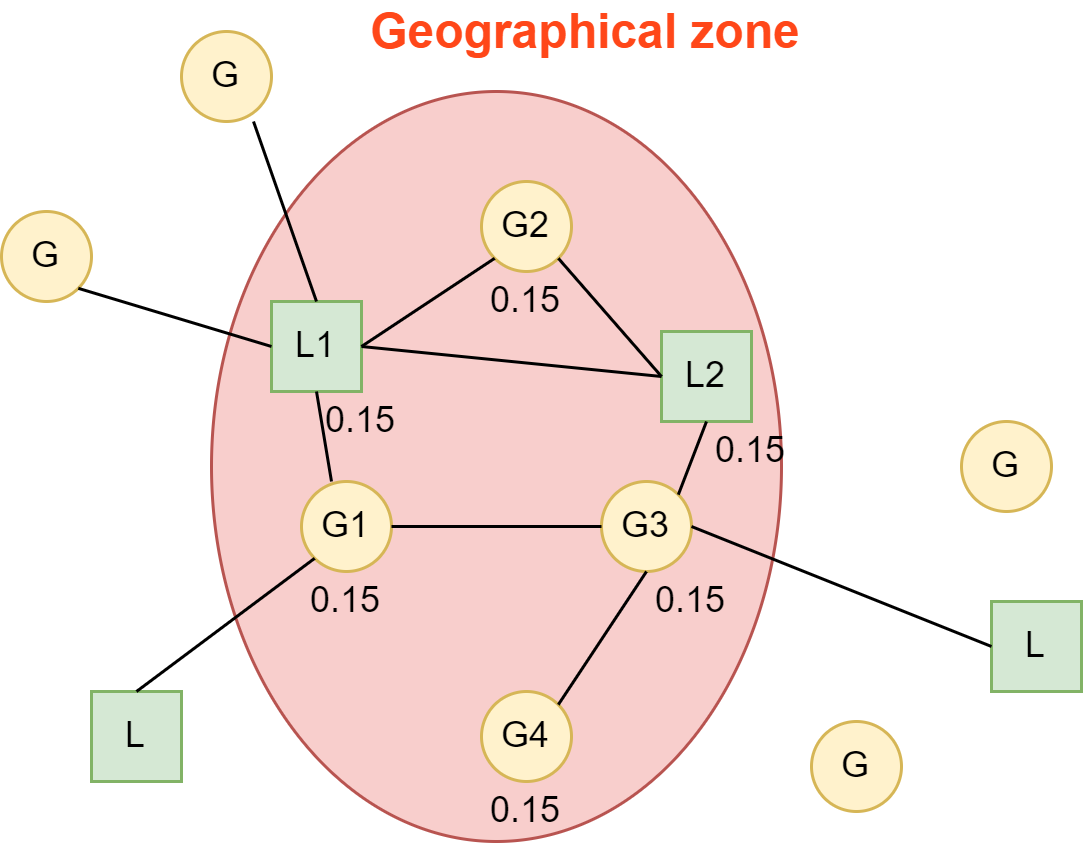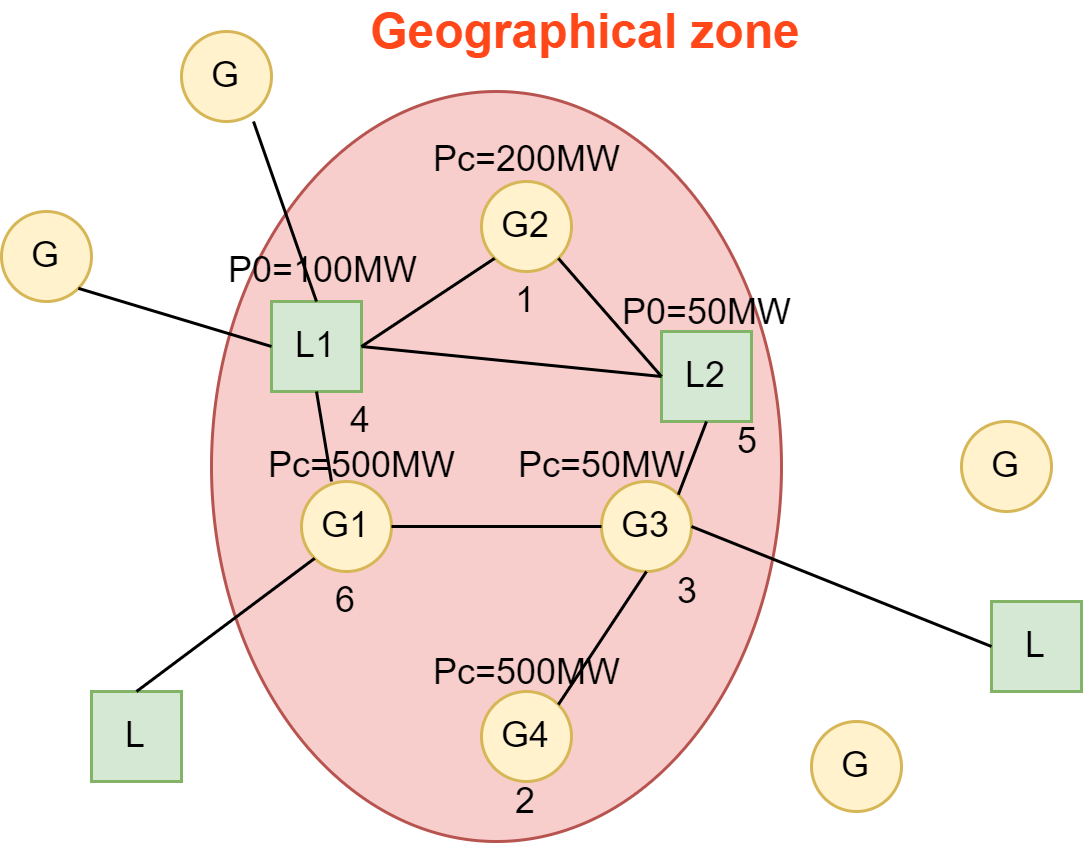# GLSK

Contents

## General definition

GLSK stands for Generators and Loads Shift Keys. The aim of this object is to define repartition keys on generators and loads of a network. It is used to apply a variation of net position on a geographical zone that could be defined as a set of generators and loads. It is used for different purposes: simple zone net position variation in a network, sensitivity computation toward a zone, loop-flow computation according to ACER definition, etc.When a variation is to be applied on the specified geographical zone – defined as a set of generators and loads within the network – the GLSK will give rules to define each variation to apply on individual generators and loads.
These rules can be different according to what is called the type of GLSK. And for the same geographical zone these rules can also be different whether the net position variation of the zone is positive or negative.

## Types of GLSK

Here we are going to talk about one unique geographical zone.

### Proportional GLSK

A proportional GLSK gives a percentage as repartition key. Within the zone, the absolute sum of these percentages must be one. Of course the variation on generators and loads must be of the opposite sign meaning that if the variation on the zone is positive generators target powers must increase and loads consumption must decrease – the aim being to increase net position.

This type of GLSK is linear therefore it can be used for PTDF computations.Let’s consider a variation of +200MW on the geographical zone, by proportionality the generators/loads will vary as follows :

• G1 +200 * 0.3 = +60MW of production
• G2 +200 * 0.1 = +20MW of production
• G3 +200 * 0.15 = +30MW of production
• G4 +200 * 0.15 = +30MW of production
• L1 +200 * 0.2 = -40MW of consumption
• L2 +200 * 0.1 = -20MW of consumption
As the sum of coefficients is 1, in the end the variation of the net position is 60+20+30+30+40+20=+200MW

⚠️ A proportional GLSK where the sum of the coefficient on a zone is not 1 will be considered invalid

Given this global definition we can define proportional GLSK in different ways.

#### Equally balanced GLSK

Given a zone, each element of the GLSK are rated equally. So there is no need to specify the coefficients, only the zone and the set of generators/loads within it is required.To define the coefficient that will be equal for all elements of the zone you divide 1 by the number of elements in the zone. Here 1 / 6 = 0.15.

#### Proportional to target power GLSK

Given a zone, each element the GLSK is rated by its participation to the net position of the zone. So there is no need to specify the coefficients, we only need to know the set of generators/loads and their associated target active power.To define the different coefficients, the sum of active powers has to be computed, here 500 + 200 + 50 + 500 + 100 + 50 = 1400MW. > Then for element the coefficient is the participation factor of this element to the net position so:

• G1 : 500 / 1400 = 0.35
• G2 : 200 / 1400 = 0.15
• G3 : 50 / 1400 = 0.035
• G4 : 500 / 1400 = 0.35
• L1 : 100 / 1400 = 0.07
• L2 : 50 / 1400 = 0.035

#### Proportional to remaining capacity

Given a zone, each element the GLSK is rated by its remaining available capacity in the direction of the variation. So there is no need to specify the coefficients, we only need to know the set of generators/loads, their associated target active power and their maximum and minimum capacity.Here for each generator and load a minimum and maximum active power have to be defined:

• G1 : 100MW - 600MW
• G2 : 50MW - 800MW
• G3 : 50MW - 200MW
• G4 : 100MW - 600MW
• L1 : 0MW - 1000MW
• L2 : 0MW - 1000MW

Let’s say we want to increase net position by 1000MW here:

• G1 : remaining capacity = 100MW
• G2 : remaining capacity = 600MW
• G3 : remaining capacity = 150MW
• G4 : remaining capacity = 100 MW
• L1 : remaining capacity = 900MW
• L2 : remaining capacity = 950MW

So the coefficients are computed toward the remaining capacity of each element:

• Sum of remaining capacity is 2800MW
• G1 : 100 / 2800 = 0.035
• G2 : 600 / 2800 = 0.214
• G3 : 150 / 2800 = 0.054
• G4 : 100 / 2800 = 0.035
• L1 : 900 / 2800 = 0.321
• L2 : 950 / 2800 = 0.339

### Merit order GLSK

A merit order GLSK gives a priority to individual generators and loads. So this type of GLSK is not linear so it cannot be used to compute PTDF and as a consequence loop-flows. It can only be used for net position variation. Merit order GLSK is an ordered list of generators and loads. To reach the variation goal the first element of the list would be shifted until it reaches its minimum or maximum value – whether it is a load or a generator and whether it is a net position increase or decrease. Once this extremum is reached the second element of the list can be shifted, and so on until the target variation is reached.Here for each generator and load a minimum and maximum active power have to be defined:

• G1 : 100MW - 600MW
• G2 : 50MW - 600MW
• G3 : 50MW - 200MW
• G4 : 100MW - 600MW
• L1 : 0MW - 1000MW
• L2 : 0MW - 1000MW

Let’s say we want to increase net position by 1000MW here:

• Start with first element, G2 that goes from 200MW to its maximum active power 600MW. That is a 400MW increase then it remains 1000 - 400 = 600MW
• Then second element, G4 that goes from 500MW to its maximum active power 600MW. That is a 100MW increase then it remains 600 - 100 = 500MW
• Then third element, G3 that goes from 50MW to its maximum active power 200MW. That is a 150MW increase then it remains 500 - 150 = 350MW
• Then the fourth element, L1 that is currently set at 100MW and that can go until 1000MW so it has a remaining capacity of 900MW which is higher that the remaining capacity to shift. So we just increase the consumption by the remaining capacity to shift, then it goes to 450MW. And the shift is achieved.

So after the shift target values are:

• G1 500MW – it didn’t change
• G2 600MW (+400MW) – its maximum power within the GLSK
• G3 200MW (+150MW) – its maximum power within the GLSK
• G4 600MW (+100MW) – its maximum power within the GLSK
• L1 450MW (+350MW)
• L2 50MW – it didn’t change